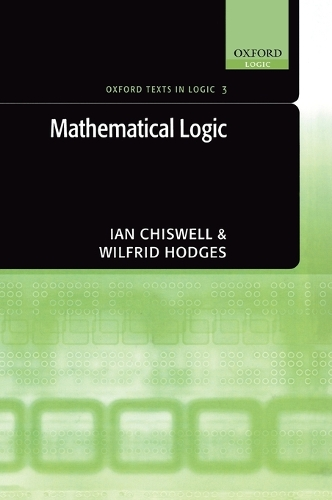•# Mathematical Logic - OXFORD TEXTS IN LOGIC 3 (Hardback)

(author), (author)
£127.50
Hardback 258 Pages / Published: 17/05/2007
• We can order this from the publisher

Usually dispatched within 15 working days

Assuming no previous study in logic, this informal yet rigorous text covers the material of a standard undergraduate first course in mathematical logic, using natural deduction and leading up to the completeness theorem for first-order logic. At each stage of the text, the reader is given an intuition based on standard mathematical practice, which is subsequently developed with clean formal mathematics. Alongside the practical examples, readers learn what can and can't be calculated; for example the correctness of a derivation proving a given sequent can be tested mechanically, but there is no general mechanical test for the existence of a derivation proving the given sequent. The undecidability results are proved rigorously in an optional final chapter, assuming Matiyasevich's theorem characterising the computably enumerable relations. Rigorous proofs of the adequacy and completeness proofs of the relevant logics are provided, with careful attention to the languages involved. Optional sections discuss the classification of mathematical structures by first-order theories; the required theory of cardinality is developed from scratch. Throughout the book there are notes on historical aspects of the material, and connections with linguistics and computer science, and the discussion of syntax and semantics is influenced by modern linguistic approaches. Two basic themes in recent cognitive science studies of actual human reasoning are also introduced. Including extensive exercises and selected solutions, this text is ideal for students in Logic, Mathematics, Philosophy, and Computer Science.

Publisher: Oxford University Press
ISBN: 9780198571001
Number of pages: 258
Weight: 643 g
Dimensions: 246 x 177 x 17 mm

MEDIA REVIEWS
Mathematical Logic is crisply written and is a pleasure to read. ..Chiswell and Hodges' book is at the very top of the reading list. * Michael Berg, MAA Online *
The text is clearly laid out and written in an easy-to-read free-flowing style. * Times Higher Education Supplement *